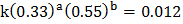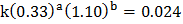Sample Problem

Consider the following reaction and experimental data:

A + B → AB

 Trial [A] (mol/L) [B] (mol/L) Rate (molL-1s-1) 1 0.66 0.55 0.096 2 0.33 0.55 0.012 3 0.33 1.10 0.024

What is the rate constant of the reaction?

Solution

First write out the rate law using the experimental data:(Trial 1)(Trial 2)(Trial 3)

To find a, divide the equation from trial 1 by the equation from trial 2 (when [B] is constant):To find b, divide the equation from trial 3 by the equation from trial 2 (when [A] is constant):Now use any equation, and plug in the experimental values:

Using trial 1Pay attention to the units of the rate constant: